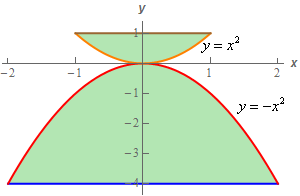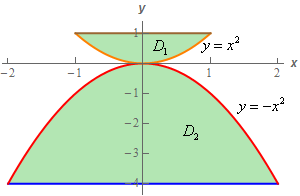Paul's Online Notes
Home / Calculus III / Multiple Integrals / Double Integrals over General Regions
Show Mobile Notice Show All Notes Hide All Notes
Mobile Notice
You appear to be on a device with a "narrow" screen width (i.e. you are probably on a mobile phone). Due to the nature of the mathematics on this site it is best views in landscape mode. If your device is not in landscape mode many of the equations will run off the side of your device (should be able to scroll to see them) and some of the menu items will be cut off due to the narrow screen width.

### Section 15.3 : Double Integrals over General Regions

7. Evaluate $$\displaystyle \iint\limits_{D}{{3 - 6xy\,dA}}$$ where $$D$$ is the region shown below.Show All Steps Hide All Steps

Start Solution

First, let’s label the two sub regions in $$D$$ as shown below.Show Step 2

Hopefully it is clear that we cannot get a single set of limits that will completely describe $$D$$ so we’ll need to split the integral as follows.

$\iint\limits_{D}{{3 - 6xy\,dA}} = \iint\limits_{{{D_{\,1}}}}{{3 - 6xy\,dA}} + \iint\limits_{{{D_{\,2}}}}{{3 - 6xy\,dA}}$ Show Step 3

The region should also (hopefully) make it clear that we’ll need to integrate $$y$$ first with both of the integrals. So, here are the limits for each integral.

$\begin{array}{*{20}{c}}\begin{array}{c}{D_{\,1}}\,\\ - 1 \le x \le 1\\ {x^2} \le y \le 1\end{array}&{\hspace{0.5in}}&\begin{array}{c}{D_{\,2}}\\ - 2 \le x \le 2\\ - 4 \le y \le - {x^2}\end{array}\end{array}$

The integrals are then,

$\iint\limits_{D}{{3 - 6xy\,dA}} = \int_{{ - 1}}^{1}{{\int_{{{x^2}}}^{1}{{3 - 6xy\,dy}}\,dx}} + \int_{{ - 2}}^{2}{{\int_{{ - 4}}^{{ - {x^2}}}{{3 - 6xy\,dy}}\,dx}}$ Show Step 4

Not much to do now other than do the integrals. Here is the $$y$$ integration for both of them.

\begin{align*}\iint\limits_{D}{{3 - 6xy\,dA}} & = \int_{{ - 1}}^{1}{{\left. {\left( {3y - 3x{y^2}} \right)} \right|_{{x^2}}^1\,dx}} + \int_{{ - 2}}^{2}{{\left. {\left( {3y - 3x{y^2}} \right)} \right|_{ - 4}^{ - {x^2}}\,dx}}\\ & = \int_{{ - 1}}^{1}{{3{x^5} - 3{x^2} - 3x + 3\,dx}} + \int_{{ - 2}}^{2}{{12 + 48x - 3{x^2} - 3{x^5}\,dx}}\end{align*} Show Step 5

Finally, here is the $$x$$ integration for both of the integrals.

\begin{align*}\iint\limits_{D}{{3 - 6xy\,dA}} & = \int_{{ - 1}}^{1}{{3{x^5} - 3{x^2} - 3x + 3\,dx}} + \int_{{ - 2}}^{2}{{12 + 48x - 3{x^2} - 3{x^5}\,dx}}\\ & = \left. {\left( {\frac{1}{2}{x^6} - {x^3} - \frac{3}{2}{x^2} + 3x} \right)} \right|_{ - 1}^1 + \left. {\left( {12x + 24{x^2} - {x^3} - \frac{1}{2}{x^6}} \right)} \right|_{ - 2}^2\\ & = \hspace{1.15in}4\hspace{1.4in} + \hspace{1.15in}32\\ & = \require{bbox} \bbox[2pt,border:1px solid black]{{36}}\end{align*}

Don’t always expect every integral over a region to be done with a single integral. On occasion you will need to split the integral up and do the actual integration over separate sub regions. In this case that was obvious but sometimes it might not be so clear until you get into the problem and realize it would be easier to do over sub regions.